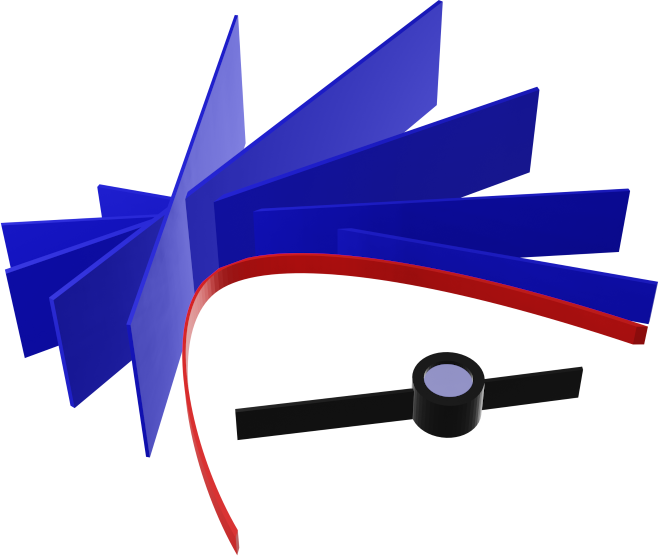# 11 November 2020

## GeoGebra Discovery: Explore envelopes easily!

One of GeoGebra's newest experimental features is the availability of the Envelope tool in the Toolbar. Now the user does not need to type the Envelope command in the Input Bar, but just to select the required tool in the Toolbar. In addition, in the latest version there is language support for Spanish, German and Hungarian to ease the user's experiments.

Let's try a simple example:
1. Create a circle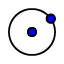with center $$A$$ and circumpoint $$B$$. The circle $$c$$ is created.
2. Put a point $$C$$ on the circle by using the Point tool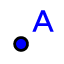.
3. Create an arbitrary point $$D$$ somewhere in the plane by using the Point tool again.
4. Create the perpendicular bisector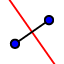of points $$C$$ and $$D$$. A line $$f$$ is created.
5. Use the Move tool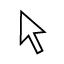to make experiments how the line $$f$$ is changing while you drag $$C$$ on the circle.
6. (Optional.) Right-click on $$f$$ and enable trace. Make some more experiments by dragging $$C$$ on the circle. When you finish this step, you may eventually disable showing trace and remove the already drawn trace by pressing Ctrl-Shift-F.
7. Use the Envelope toolto select line $$f$$ and the mover point $$C$$. This results in showing the envelope of the lines $$f$$ while $$C$$ is moving on its path.
8. (Optional.) Select the created object $$eq1$$ and change its color to make a difference to the other objects. Eventually you can try steps 5 and 6 again to visually check if the envelope is indeed always touched by all possible lines $$f$$.
9. Change point $$D$$ to learn how the geometric properties of the envelope depend on your selection.
Acknowledgment. The introductory figure is a contribution of my son Benedek. He used the SVG version of the Envelope tool icon, and imported it in Blender, then, after some magick, he rendered a 3D view figure and voilà!Zoltán Kovács Linzer Zentrum für Mathematik Didaktik Johannes Kepler Universität Altenberger Strasse 54 A-4040 Linz